# DERIVATION OF THE RADIX-2 FFT ALGORITHM

This section and those that follow provide a detailed description of the internal data structures and operations of the radix-2 FFT for those readers interested in developing software FFT routines or designing FFT hardware. To see just exactly how the FFT evolved from the DFT, we return to the equation for an N-point DFT,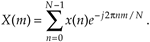A straightforward derivation of the FFT proceeds with the separation of the input data sequence x(n) into two parts. When x(n) is segmented into its even and odd indexed elements, we can, then, break Eq. (4-11) into two parts as

Equation 4-12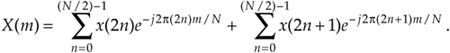Pulling the constant phase angle outside the second summation,

Equation 4-13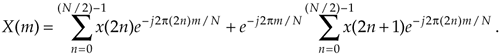Well, here the equations get so long and drawn out that we'll use the standard notation to simplify things. We'll define WN = e–j2p/N to represent the complex phase angle factor that is constant with N. So Eq. (4-13) becomes

Equation 4-14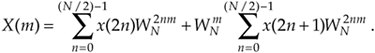Because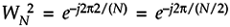, we can substitutein Eq. (4-14), as

Equation 4-15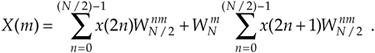So we now have two N/2 summations whose results can be combined to give us the N-point DFT. We've reduced some of the necessary number crunching in Eq. (4-15) relative to Eq. (4-11) because the W terms in the two summations of Eq. (4-15) are identical. There's a further benefit in breaking the N-point DFT into two parts because the upper half of the DFT outputs is easy to calculate. Consider the X(m+N/2) output. If we plug m+N/2 in for m in Eq. (4-15), then

Equation 4-16It looks like we're complicating things, right? Well, just hang in there for a moment. We can now simplify the phase angle terms inside the summations because

Equation 4-17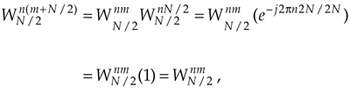for any integer n. Looking at the so-called twiddle factor in front of the second summation in Eq. (4-16), we can simplify it as

Equation 4-18OK, using Eqs. (4-17) and (4-18), we represent Eq. (4-16)'s X(m+N/2) as

Equation 4-19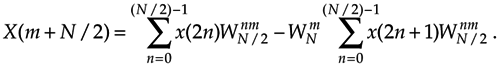Now, let's repeat Eqs. (4-15) and (4-19) to see the similarity;

Equation 4-20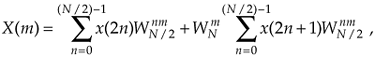and

Equation 4-20'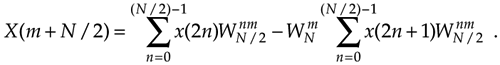So here we are. We need not perform any sine or cosine multiplications to get X(m+N/2). We just change the sign of the twiddle factor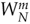and use the results of the two summations from X(m) to get X(m+N/2). Of course, m goes from 0 to (N/2)–1 in Eq. (4-20) which means, for an N-point DFT, we perform an N/2-point DFT to get the first N/2 outputs and use those to get the last N/2 outputs. For N = 8, Eqs. (4-20) and (4-20') are implemented as shown in Figure 4-2.

Figure 4-2. FFT implementation of an 8-point DFT using two 4-point DFTs.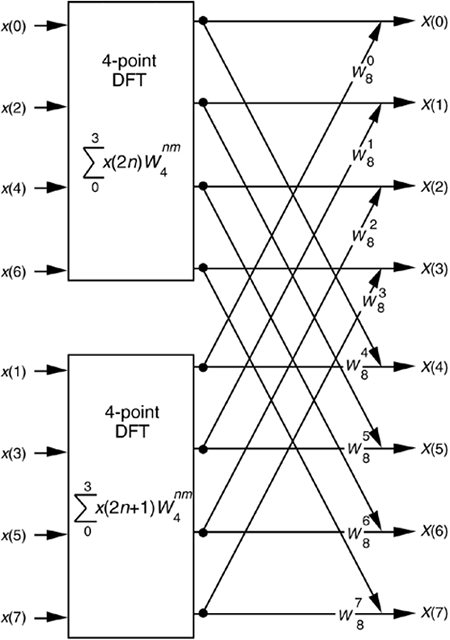If we simplify Eqs. (4-20) and (4-20') to the form

Equation 4-21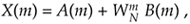and

Equation 4-21'we can go further and think about breaking the two 4-point DFTs into four 2-point DFTs. Let's see how we can subdivide the upper 4-point DFT in Figure 4-2 whose four outputs are A(m) in Eqs. (4-21) and (4-21'). We segment the inputs to the upper 4-point DFT into their odd and even components

Equation 4-22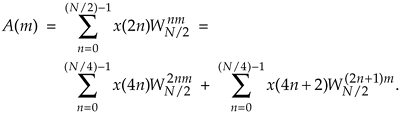Because, we can express A(m) in the form of two N/4-point DFTs, as

Equation 4-23Notice the similarity between Eq. (4-23) and Eq. (4-20). This capability to subdivide an N/2-point DFT into two N/4-point DFTs gives the FFT its capacity to greatly reduce the number of necessary multiplications to implement DFTs. (We're going to demonstrate this shortly.) Following the same steps we used to obtained A(m), we can show that Eq.(4-21)'s B(m) is

Equation 4-24For our N = 8 example, Eqs. (4-23) and (4-24) are implemented as shown in Figure 4-3. The FFT's well-known butterfly pattern of signal flows is certainly evident, and we see the further shuffling of the input data in Figure 4-3. The twiddle factor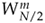in Eqs. (4-23) and (4-24), for our N = 8 example, ranges from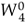to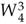because the m index, for A(m) and B(m), goes from 0 to 3. For any N-point DFT, we can break each of the N/2-point DFTs into two N/4-point DFTs to further reduce the number of sine and cosine multiplications. Eventually, we would arrive at an array of 2-point DFTs where no further computational savings could be realized. This is why the number of points in our FFTs are constrained to be some power of 2 and why this FFT algorithm is referred to as the radix-2 FFT.

Figure 4-3. FFT implementation of an 8-point DFT as two 4-point DFTs and four 2-point DFTs.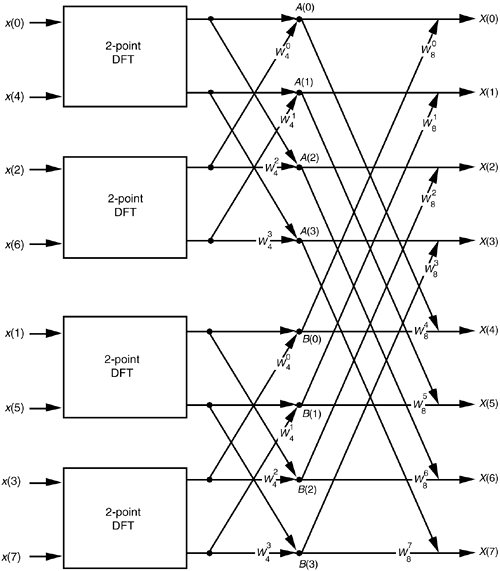Moving right along, let's go one step further, and then we'll be finished with our N = 8 point FFT derivation. The 2-point DFT functions in Figure 4-3 cannot be partitioned into smaller parts—we've reached the end of our DFT reduction process arriving at the butterfly of a single 2-point DFT as shown in Figure 4-4. From the definition of WN'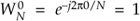and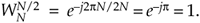. So the 2-point DFT blocks in Figure 4-3 can be replaced by the butterfly in Figure 4-4 to give us a full 8-point FFT implementation of the DFT as shown in Figure 4-5.

Figure 4-4. Single 2-point DFT butterfly.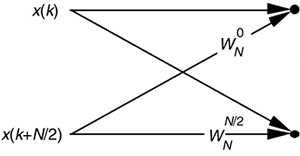Figure 4-5. Full decimation-in-time FFT implementation of an 8-point DFT.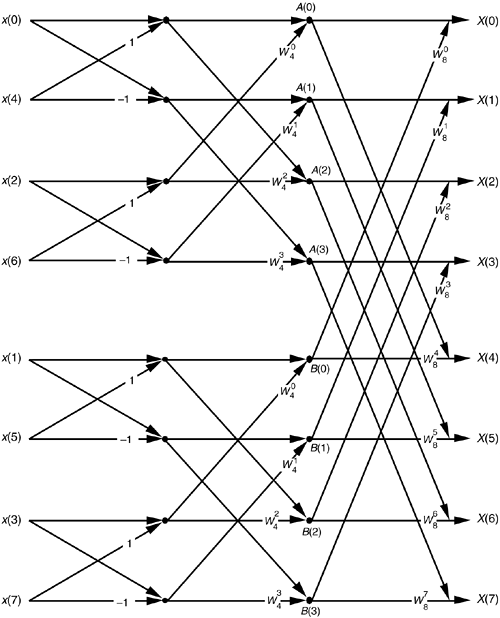OK, we've gone through a fair amount of algebraic foot shuffling here. To verify that the derivation of the FFT is valid, we can apply the 8-point data sequence of Chapter 3's DFT Example 1 to the 8-point FFT represented by Figure 4-5. The data sequence representing x(n) = sin(2p1000nts) + 0.5sin(2p2000nts+3p/4) is

Equation 4-25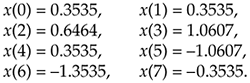We begin grinding through this example by applying the input values from Eq. (4-25) to Figure 4-5, giving the data values shown on left side of Figure 4-6. The outputs of the second stage of the FFT are

Figure 4-6. 8-point FFT of Example 1 from Section 3.1.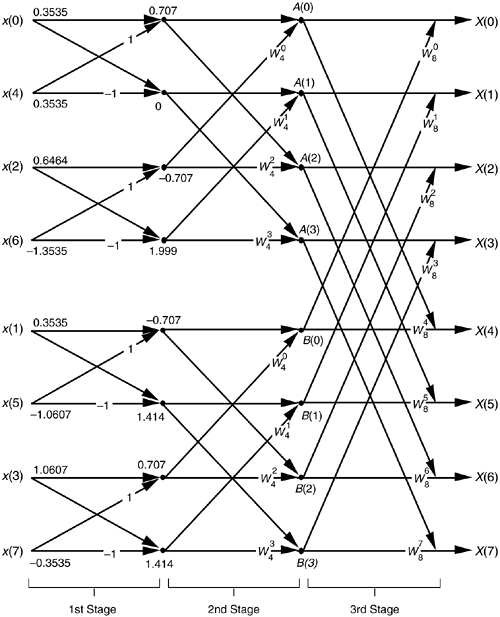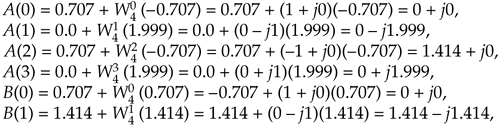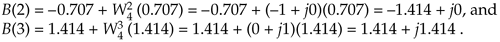Calculating the outputs of the third stage of the FFT to arrive at our final answerSo, happily, the FFT gives us the correct results, and again we remind the reader that the FFT is not an approximation to a DFT; it is the DFT with a reduced number of necessary arithmetic operations. You've seen from the above example that the 8-point FFT example required less effort than the 8-point DFT Example 1 in Section 3.1. Some authors like to explain this arithmetic reduction by the redundancies inherent in the twiddle factors. They illustrate this with the starburst pattern in Figure 4-7 showing the equivalencies of some of the twiddle factors in an 8-point DFT.

Figure 4-7. Cyclic redundancies in the twiddle factors of an 8-point FFT.URL http://proquest.safaribooksonline.com/0131089897/ch04lev1sec4Amazon